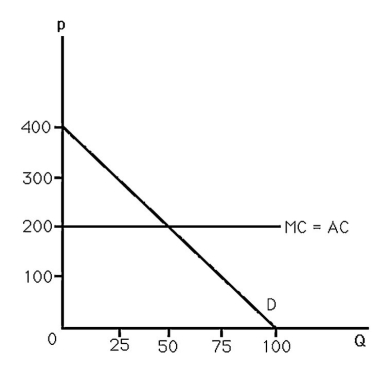# Globale Microeconomics

## Quiz 11 : MonopolyStudy FlashcardsLooking for Economics Homework Help?

## Quiz 11 :Monopoly

Question TypeAt the current level of output, a firm's marginal cost equals 16 and marginal revenue equals 10. The firm
Free
Multiple Choice

C

Tags
Choose question tagA profit-maximizing monopolist will never operate in the portion of the demand curve with price elasticity equal to
Free
Multiple Choice

C

Tags
Choose question tag-The above figure shows the demand and cost curves facing a monopoly. The monopoly maximizes profit by selling
Free
Multiple Choice

B

Tags
Choose question tagThe monopoly maximizes profit by setting
Multiple Choice
Tags
Choose question tagAt an output level of 100, a monopolist faces MC = 15 and MR = 17. At output level q = 101, the monopolist's MC = 16 and MR = 15. To maximize profits, the firm
Multiple Choice
Tags
Choose question tagFor a monopoly, marginal revenue is less than price because
Multiple Choice
Tags
Choose question tagIf the inverse demand curve a monopoly faces is p = 100 - 2Q, and MC is constant at 16, then profit maximization
Multiple Choice
Tags
Choose question tag-The above figure shows the demand and cost curves facing a monopolist. The monopoly maximizes profit by setting price equal to
Multiple Choice
Tags
Choose question tag-The above figure shows the demand and cost curves facing a monopoly. Maximum profit equals
Multiple Choice
Tags
Choose question tagIf the inverse demand curve a monopoly faces is p = 100 - 2Q, and MC is constant at 16, then profit maximization is achieved when the monopoly sets price equal to
Multiple Choice
Tags
Choose question tagOne difference between a monopoly and a competitive firm is that
Multiple Choice
Tags
Choose question tagFor a monopoly, marginal revenue is less than price because
Multiple Choice
Tags
Choose question tagIf the inverse demand curve a monopoly faces is p = 100 - 2Q, and MC is constant at 16, then maximum profit
Multiple Choice
Tags
Choose question tagIf the inverse demand curve a monopoly faces is p = 100 - 2Q, then profit maximization
Multiple Choice
Tags
Choose question tagIf a firm is able to influence its price,
Multiple Choice
Tags
Choose question tagA monopolist faces the inverse demand curve P = 60 - Q. It has variable costs of Q2 so that its marginal costs are 2Q, and it has fixed costs of 30. The monopoly's profit-maximizing output is
Multiple Choice
Tags
Choose question tagIf the inverse demand function for a monopoly's product is p = a - bQ, then the firm's marginal revenue function is
Multiple Choice
Tags
Choose question tagA monopolist faces the inverse demand curve P = 60 - Q. It has variable costs of Q2 so that its marginal costs are 2Q, and it has fixed costs of 30. The monopoly's profit-maximizing price is
Multiple Choice
Tags
Choose question tagMarginal Revenue is
Multiple Choice